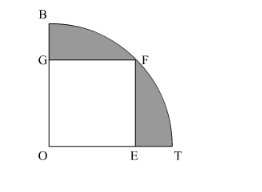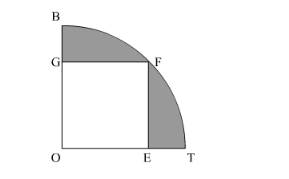# In the following figure, OE = 20 cm. In sector OSFT,Question:

In the following figure, OE = 20 cm. In sector OSFT, square OEFG is inscribed. Find the area  of the shaded region.Solution:

We have to find the area of the shaded portion. We have, $\mathrm{OE}=20 \mathrm{~cm}$ and $\mathrm{OEFG}$ is a square.Use Pythagoras theorem to find OF as,

$\mathrm{OF}=\left(\sqrt{(20)^{2}+(20)^{2}}\right) \mathrm{cm}$

$=20 \sqrt{2} \mathrm{~cm}$

Therefore area of the shaded region,

Area of the shaded region $=($ Area of quadrant of cicle $)-($ Area of square $)$

So,

Area of the shaded region $=\frac{\pi(\mathrm{OF})^{2}}{4}-(\mathrm{OE})^{2}$

$=\left(\frac{22}{7}\right)\left(\frac{(20 \sqrt{2})^{2}}{4}\right)-(20)^{2}$

$=628-400$

$=228 \mathrm{~cm}^{2}$